ImpulseBy Wikipedia,
the free encyclopedia,

http://en.wikipedia.org/wiki/Impulse

In classical mechanics, an impulse is defined as the integral of a force with respect to time. When a force is applied to a rigid body it changes the momentum of that body. A small force applied for a long time can produce the same momentum change as a large force applied briefly, because it is the product of the force and the time for which it is applied that is important.

## Mathematical derivation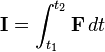$\mathbf{I} = \int_{t_1}^{t_2} \mathbf{F}\, dt$

where

I is impulse (frequently marked J),
F is the force, and
dt is an infinitesimal amount of time.
t1 and t2 denote a time interval

A simple derivation using Newton's second law yields: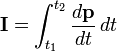$\mathbf{I} = \int_{t_1}^{t_2} \frac{d\mathbf{p}}{dt}\, dt$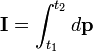$\mathbf{I} = \int_{t_1}^{t_2} d\mathbf{p}$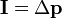$\mathbf{I} = \Delta \mathbf{p}$

where

p is momentum

This is often called the impulse-momentum theorem.

As a result, an impulse may also be regarded as the change in momentum of an object to which a force is applied. The impulse may be expressed in a simpler form when both the force and the mass are constant: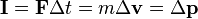$\mathbf{I} = \mathbf{F}\Delta t = m \Delta \mathbf{v} = \Delta\mathbf{p}$

where

F is the constant total net force applied,
Δt is the time interval over which the force is applied,
m is the constant mass of the object,
Δv is the change in velocity produced by the force in the considered time interval, and
v = Δ(mv) is the change in linear momentum.

It is often the case that not just one but both of these two quantities vary.

In the technical sense, impulse is a physical quantity, not an event or force. The term "impulse" is also used to refer to a fast-acting force. This type of impulse is often idealized so that the change in momentum produced by the force happens with no change in time. This sort of change is a step change, and is not physically possible. This is a useful model for certain purposes, such as computing the effects of ideal collisions, especially in game physics engines.

Impulse has the same units and dimensions as momentum (kg m/s = N·s).

Impulse can be calculated using the equation: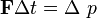$\mathbf{F}\Delta t = \Delta\ p$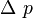$\Delta\ p$ can be calculated, if initial and final velocities are known,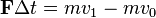$\mathbf{F}\Delta t = mv_1 - mv_0$

where

F is the constant total net force applied,
t is the time interval over which the force is applied,
m is the constant mass of the object,
v1 is the final velocity of the object at the end of the time interval, and
v0 is the initial velocity of the object when the time interval begins.

## Bibliography

• Serway, Raymond A.; Jewett, John W. (2004). Physics for Scientists and Engineers (6th ed. ed.). Brooks/Cole. ISBN 0-534-40842-7.
• Tipler, Paul (2004). Physics for Scientists and Engineers: Mechanics, Oscillations and Waves, Thermodynamics (5th ed. ed.). W. H. Freeman. ISBN 0-7167-0809-4.

Published in July 2009.